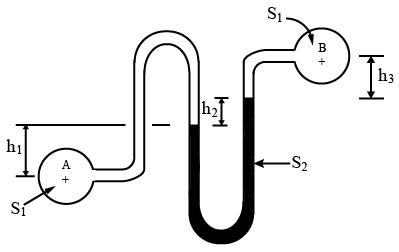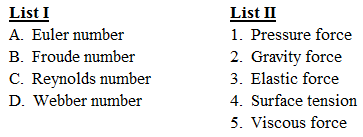# ISRO Scientist or Engineer Mechanical 2006

Instructions

For the following questions answer them individually

Question 61

# The actual velocity at vena contracta for flow through an orifice from a reservoir of height H =Question 62

# The horizontal component of force on a curved surface is equal to theQuestion 63

# The maximum depth from which a centrifugal pump can draw water isQuestion 64

# Two pipe lines at a different pressures, $$p_A$$ and $$p_B,$$ each carrying the same liquid of specific gravity $$S_1$$ are connected to a U tube with a liquid of specific gravity of $$S_2$$ resulting in the level differences $$h_1, h_2 and h_3$$ as shown in the figure. The difference in pressure head between points A and B in terms of head of water isQuestion 65

# List I gives 4 dimensionless numbers andList II gives the types of forces, which are one of the constituents describing the numbers. Match list I with List II and select the correct answer using the codes given below the lists:Question 66

# A single-stage impulse turbine with a diameter of 120 cm runs at 3000 rpm. If the blade speed ratio is 0.42, then, the inlet velocity of steam will beQuestion 67

# For laminar flow over a flat plate, the thickness of the boundary layer at a distance from the leading edge is found to be 5 mm. The thickness of the boundary layer at a downstream section, which is at twice the distance of the previous section from the leading edge, will beQuestion 68

# If $$U_\infty$$ = free stream velocity, u = velocity at y, and $$\delat = boundary layer thickness, then in a boundary layer flow, the momentum thickness$$\theta is given byQuestion 69

# An automobile moving at a velocity of 40 km/hr is experiencing a wind resistance of 2kN. If the automobile is moving at a velocity of 50km/hr, the power required to overcome the wind resistance isQuestion 70

# A cons tant-head water tank has, on one of its vertical sides two identical small orifices issuing two horizontal jets in the same vertical plane. The vertical distance between the centers of orifices is 1.5 m and the jet trajectories intersect at a point 0.5 m below the lower orifice. What is the approximate height of water level in the tank above the point of intersection trajectories?OR